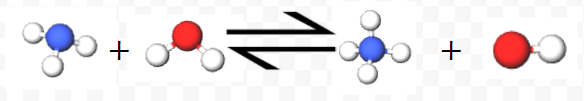# 11.11: Weak Bases

By analogy with weak acids, weak bases are not strong enough proton acceptors to react completely with water. A typical example is ammonia, which reacts only to a limited extent:

$\text{NH}_{3} + \text{H}_{2}\text{O} \rightleftharpoons \text{NH}_{4}^{+} + \text{OH}^{-}$As in the case of acetic acid, the current conducted by 0.001 M NH3 (Table 1 from Ions in Solution) is slightly above 10 percent that of 0.001 M NaOH or KOH, indicating that somewhat more than one-tenth of the NH3 molecules have been converted to NH4+ and OH ions.

To verify that NH3 is a weak base, a litmus test can be done. The NH3 is added to litmus paper, which indicates it's pH based on the color of the litmus paper. In the image below you can see the litmus paper turns a greenish blue when exposed to NH3 (pH 9-10). For comparison, a strong base of the same molarity like NaOH or KOH would have a darker blue coloring.Weak bases fall into two main categories.

Ammonia and amines

Amines may be derived from ammonia by replacing one or more hydrogens with carbon chains. They react with water in the same way as ammonia. Trimethyl amine behaves as follows:

$(\text{CH}_{3})_{3}\text{N} + \text{H}_{2}\text{O} \rightleftharpoons (\text{CH}_{3})_{3}\text{NH}^{+} + \text{OH}^{-}$ Anions of weak acids

The fact that the molecules of a weak acid are not entirely converted into hydronium ions and anions implies that those anions must have considerable affinity for protons. For example, the anion of acetic acid, acetate ion, can accept protons as follows:

$\text{C}_{2}\text{H}_{3}\text{O}_{2}^{-} + \text{H}_{2}\text{O} \rightleftharpoons \text{HC}_{2}\text{H}_{3}\text{O}_{2} + \text{OH}^{-}$

Thus when a compound like sodium acetate,dissolves, some hydroxide ions are produced and the solution becomes slightly basic. (Sodium ions do not react with water at all, and so they have no effect on acidity or basicity.) Other examples of weak bases which are anions of weak acids are CN, CO32–, PO43–.